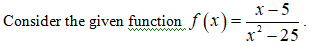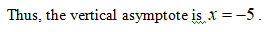# Consider the following.f(x) = x − 5x2 − 25  Find any vertical and horizontal asymptotes. (Enter your answers as a comma-separated list of equations. If there are no asymptotes, enter DNE.)

Question
28 views
Consider the following.
f(x) =
 x − 5 x2 − 25

Find any vertical and horizontal asymptotes. (Enter your answers as a comma-separated list of equations. If there are no asymptotes, enter DNE.)

check_circle

Step 1Step 2

Simplify the given function as follows.

Step 3

For rational functions the vertical asymptotes are the undefined points, that is equate the denominator of the simplified function to zero....

### Want to see the full answer?

See Solution

#### Want to see this answer and more?

Solutions are written by subject experts who are available 24/7. Questions are typically answered within 1 hour.*

See Solution
*Response times may vary by subject and question.
Tagged in

### Functions